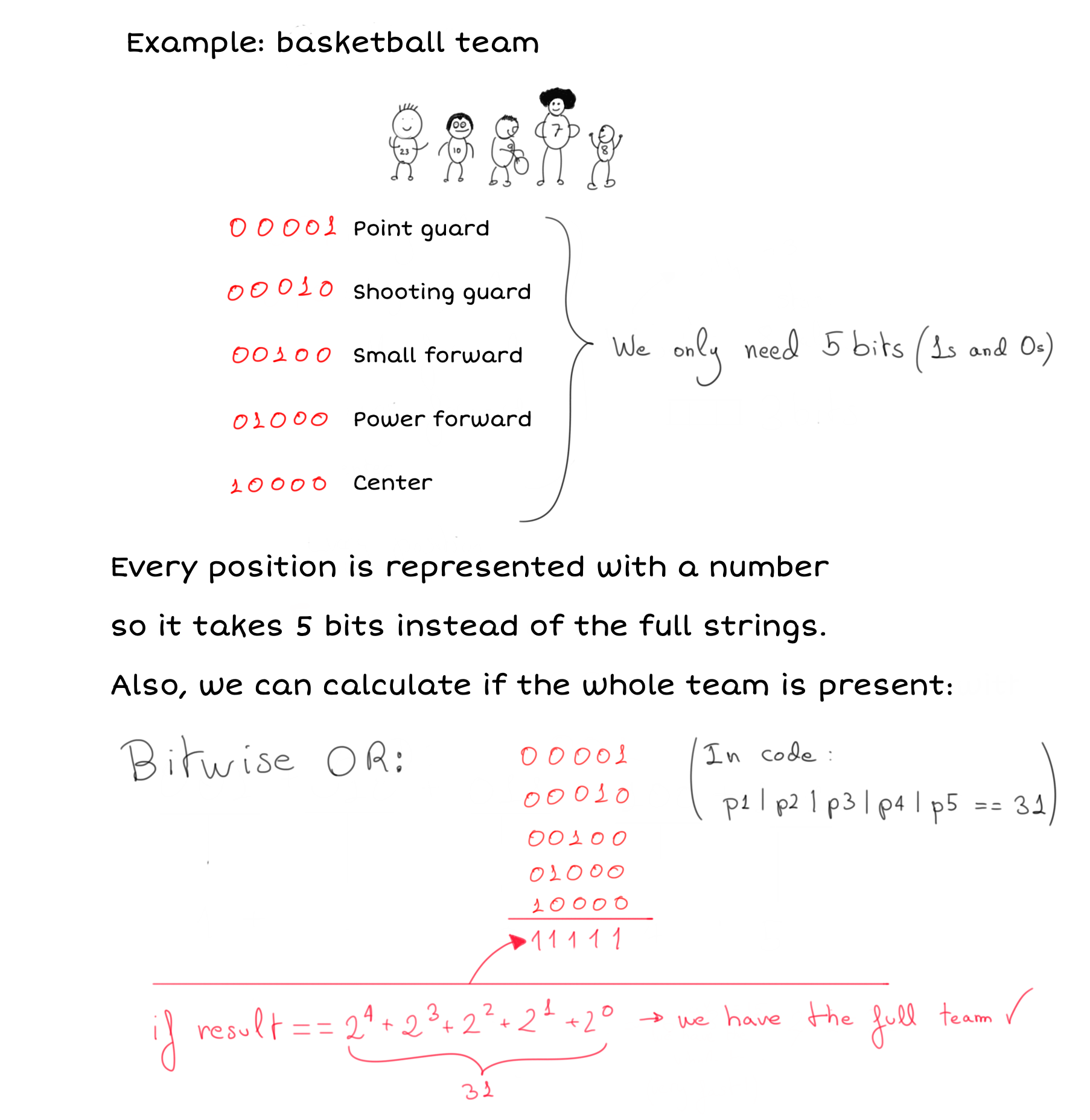Part of the series: Computer Science Basics

Bitwise operations are really useful and fast for storing and operate on certain values. They are way more used in systems where resources are more limited like video-games or embedded devices.

In this first article about bitwise operations, we get a quick introduction to them with a drawing (new format!) and some written content but I plan on sharing more 🙂.

## What are bitwise operations?

They’re operations that deal with bits directly. Bits are 1s and 0s and it’s how everything is stored internally in a computer so being able to operate with them directly is very efficient because the processor is able to do those operations natively. Wikipedia says:

…typically, bitwise operations are substantially faster than division, several times faster than multiplication, and sometimes significantly faster than addition…

## The three basic operators

There are more but let’s take a look today at the three most basic operators:

### NOT

NOT is very simple, if there’s a 1 we get 0. If there’s a 0, we get 1.

It’s represented by `~` in most programming languages so for example: `~0101011 = 1010100`.

### AND

AND is like the boolean equivalent you already use in if conditions but with one difference, this operates at the level of bits:

``````1 & 1 = 1
1 & 0 = 0
0 & 1 = 0
0 & 0 = 0
``````

### OR

The same goes for the OR operation, represented with the | symbol in many programming languages.

``````1 | 1 = 1
1 | 0 = 1
0 | 1 = 1
0 | 0 = 0
``````

## How they operate with more than 1 bit

The key here is to remember that when we have a bitwise operation with more than 1 bit, we do it bit by bit. For example:

``````// Bitwise OR
111010
001100
-------
111110
``````

Or in an AND operation:

``````// Bitwise AND
111010
001100
-------
001000
``````

## Example to show how to use them:Full drawing here